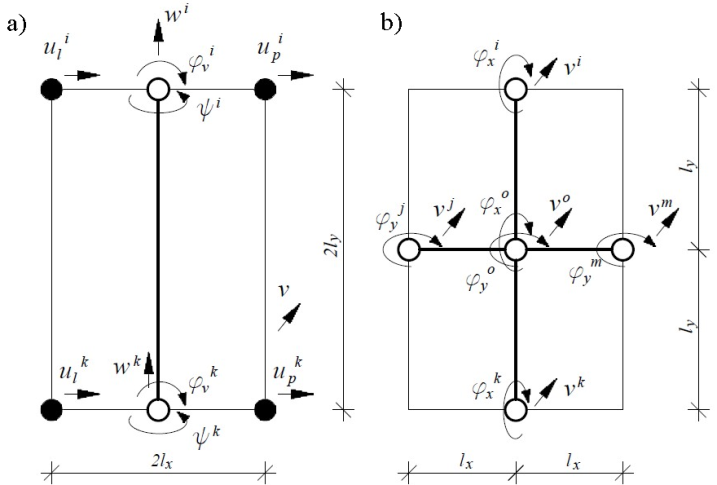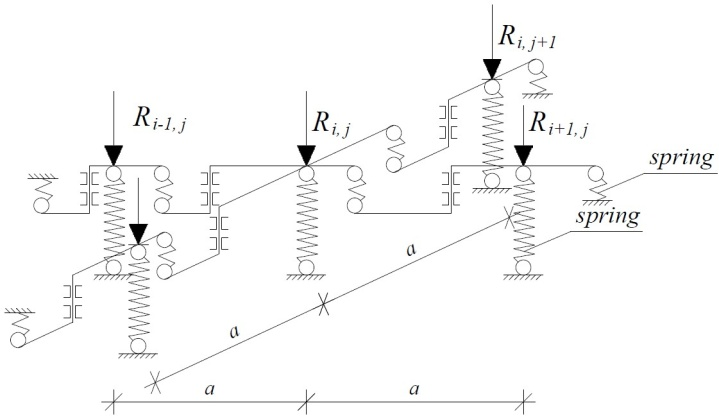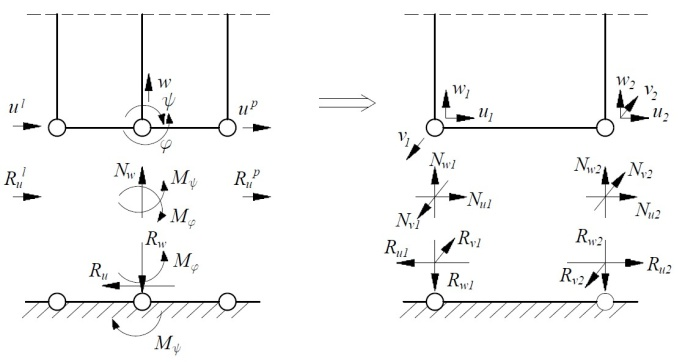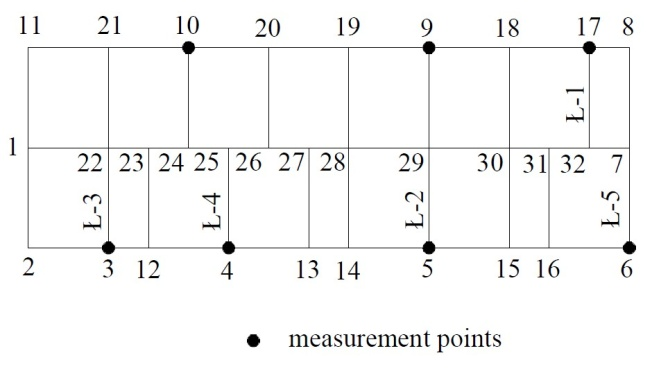## Figures index

#### Czeslaw Miedzialowski, Joanna Kretowska

American Journal of Civil Engineering and Architecture. 2014, 2(4), 143-148 doi:10.12691/ajcea-2-4-4• Figure 1. The building structure and loads scheme• Figure 2. Shield state and plate state of building structure elements: a) shield state, b) plate state• Figure 3. Finite element which describes shield and plate state• Figure 4. Three-dimensional joint model a) interconnections between 8-node spatial joint element and wall elements, b) 8-node spatial joint element• Figure 5. Graph of the shape function N3• Figure 6. Model of the approximated elastic half-space• Figure 7. Soil.-structure interaction system• Figure 8. Analyzed computational schemes• Figure 9. The horizontal projection of the building and the measuring points location• Figure 10. Diagrams of analyzed building settlement